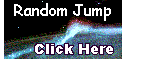Index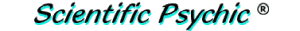# Estimate of the water ejected by an extraterrestrial impact on a glacier ice sheet

Secondary impacts by ice ejected from an extraterrestrial impact on the ice sheet over Michigan produced inclined conical cavities that transformed into the elliptical Carolina Bays.

The energy of the extraterrestrial impact was estimated to be approximately 6.35 × 1021 Joules based on the number of bays and the energy required to create them (Zamora 2013). However, the total energy of the extraterrestrial impact should also include the energy to produce the liquid ejecta associated with the impact. Here we evaluate a method for estimating the amount of water ejected by such an impact.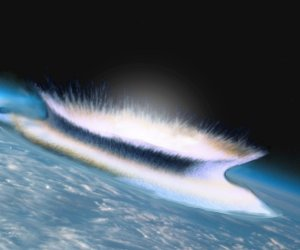A hyperspeed impact is a very energetic event. An extraterrestrial impact on a rocky surface generates a lot of heat which melts, vaporizes and ejects pieces of rock. Something similar happens with ice. A hypervelocity impact on ice produces heat that melts and vaporizes great quantities of ice, but since ice is a bad conductor of heat and very brittle, many ice fragments are ejected from the impact site. The ejecta would consist not only of pieces of ice, but also liquid water accelerated by steam at high pressure. Although the creation of the Carolina Bays could have occurred at any time during the ice ages in North America, the late Pleistocene Epoch, 12,900 years ago, provides the best fit. This is the time of the onset of the Younger Dryas cooling event, the time of the extinction of the North American megafauna, and the disappearance of the Clovis culture.

The Younger Dryas stadial was a period of cold climatic conditions that started abruptly 12,900 years ago and lasted for 1300 years. Although the calculation of the amount of water ejected by an extraterrestrial impact during the late Pleistocene may appear impossible, some estimates may be made by assuming that the Younger Dryas stadial was caused by blockage of the light of the Sun by ice particles that were formed when water was ejected above the atmosphere.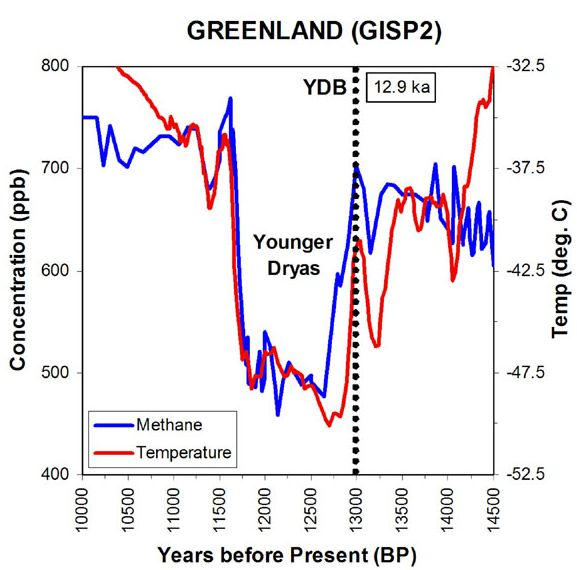The amount of ice particles in low Earth orbit can be estimated based on the rate of sublimation of ice and the duration of the cold event. Once the amount of ice is known, the amount of water ejected and the energy to melt the glacier ice can be calculated. The size of the extraterrestrial object derived from these calculations can then be used to constrain the results to a reasonable estimate.

The standard equation for the evaporation or sublimation rate from a planar surface of pure water or ice in a vacuum is given by S0 (Andreas 2007), where S0 is a mass flux; its units are kg m−2 s−1. Also, esat,i(T) is the saturation vapor pressure (in pascals) for a planar ice surface at temperature T (in kelvins), Mw is the molecular weight of water (18.015×10−3 kg mol−1), and R is the universal gas constant (8.31447 J mol−1 K−1).

$$S_0 = e_{sat,i}(T)\left(M_w \over 2 \pi RT \right)$$

The equation for esat,i(T) provided by Murphy and Koop (2005) for T in [110, 273.15 K] calculates esat,i(T) in Pa, and T must be in kelvins.

esat,i(T) = exp(9.550426 − 5723.265/T + 3.53068 ln(T) − 0.00728332T);

Assuming that the ice crystals had a surface area equivalent to the surface of a sphere 100 kilometers above the Earth's surface, and given that the Earth's radius is 6371 km, the kilograms of ice crystals can be obtained by multiplying the sublimation rate S0 times the area and the duration of the Younger Dryas stadial (1300 years). The amount of water ejected can be based on the thermodynamics for converting water into ice; approximately 80% of the water turns into ice crystals. The estimated amount of water depends on the exposed area and the time elapsed, but the temperature has the biggest influence on the result. The following graph shows the amount of water estimated as a function of temperature.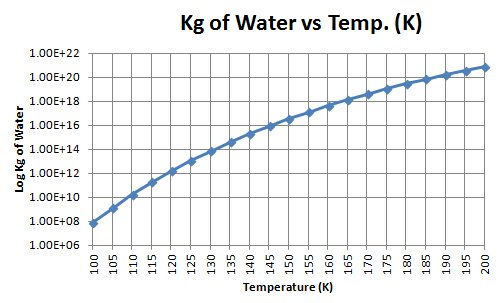Amount of water based on temperature

The estimates of the amount of water ejected can be used to calculate the energy required to melt enough glacier ice to create the water. A velocity of approximately 3.5 km/sec is required to launch the water into low Earth orbit (~150 km). The energy required to launch the water can be obtained from the formula for kinetic energy Ke=1/2(mv2). The energy to produce and launch the water, added to the energy calculated to launch the ice boulders (6.35 × 1021) would be approximately the energy of the extraterrestrial impact.

The following table shows the total energy required to launch the water and the ice. The total energy is correlated with the size of an asteroid traveling at 17 km/sec. The size of the asteroid increases very rapidly for quantities of water exceeding 2 × 1015 kg. It is unlikely that an asteroid exceeding 4 km in diameter could have been responsible for the creation of the Carolina bays because such a large asteroid would have left a visible crater on the surface of the Earth even if it was covered by an ice sheet with a thickness of one kilometer. A more fragile icy comet with a diameter of 3 km traveling at 50 km/sec would have had the same energy but would have been less likely to leave a trace of the collision under such a thick ice sheet.In modern times, the temperature at an altitude of 100 kilometers is approximately 200°K, and it increases with altitude into the thermosphere. The table above indicates that reasonable numbers can only be obtained if the temperature at such altitude could have been 60 degrees lower during the Younger Dryas stadial than today. This may have been possible, considering that the Earth would have had increased albedo from the orbiting ice crystal clouds that blocked the light of the Sun.